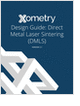MaterialsDesign CenterProcessesUnit ConversionFormulasMathematicsCalculatorsDiscussion ForumTrade PublicationsDirectory Service

Learn the best principles to negotiate the salary you deserve!

3D Scanners

A white paper to assist in the evaluation of 3D scanning hardware solutions.

Salary Expectation

8 things to know about the interview question "What's your salary expectation"?

Metal 3D Printing Design Guide

Direct Metal Laser Sintering (DMLS) 3D printing for parts with reduced cost and little waste.

more free magazinesGlossary » Units » Electric Current Density » Ampere Per Square DecimeterAmpere Per Square Decimeter (A/dm2) is a unit in the category of Electric current density. It is also known as amperes per square decimeter, ampere per square decimetre, amperes per square decimetre, ampere/square decimeter, ampere/square decimetre. This unit is commonly used in the mixed unit system. Ampere Per Square Decimeter (A/dm2) has a dimension of L-2I where L is length, and I is electric current. It can be converted to the corresponding standard SI unit A/m2 by multiplying its value by a factor of 100.
Note that the seven base dimensions are M (Mass), L (Length), T (Time), Q (Temperature), N (Aamount of Substance), I (Electric Current), and J (Luminous Intensity).

Other units in the category of Electric current density include Abampere Per Square Centimeter (aA/cm2), Ampere Per Square Centimeter (A/cm2), Ampere Per Square Foot (A/ft2), Ampere Per Square Inch (A/in2), Ampere Per Square Meter (A/m2), Coulomb Per Second Per Square Meter (C/s-m2), and Milliampere Per Square Centimeter (mA/cm2).N/A
Related PagesGlossaryLearn the best principles to negotiate the salary you deserve!3D Scanners

A white paper to assist in the evaluation of 3D scanning hardware solutions.Salary Expectation

8 things to know about the interview question "What's your salary expectation"?Metal 3D Printing Design Guide

Direct Metal Laser Sintering (DMLS) 3D printing for parts with reduced cost and little waste.